## RD Sharma Class 8 Solutions Chapter 2 Powers Ex 2.1

These Solutions are part of RD Sharma Class 8 Solutions. Here we have given RD Sharma Class 8 Solutions Chapter 2 Powers Ex 2.1

Other Exercises

Question 1.
Express each of the following as a rational number of the form $$\frac { p }{ q } ,$$ where p and q are integers and q≠ 0 :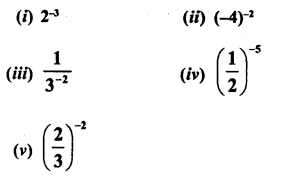Solution: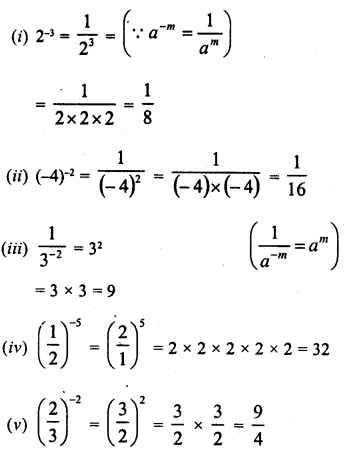Question 2.
Find the values of each of the following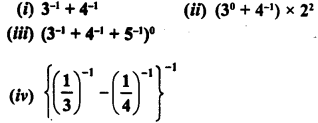Solution: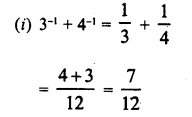Question 3.
Find the values of each of the following :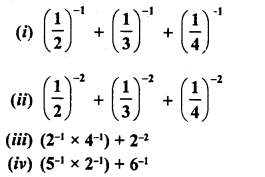Solution: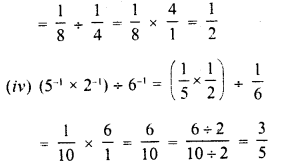Question 4.
Simplify :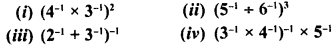Solution:Question 5.
Simplify :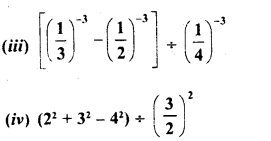Solution: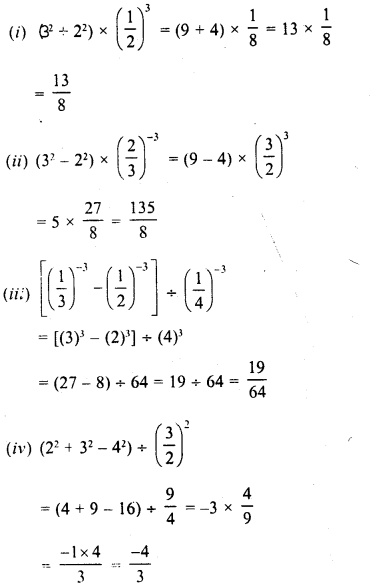Question 6.
By what number should 5-1 be multiplied so that the product may be equal to(-7)-1 ?
Solution: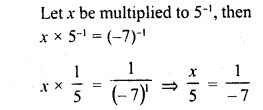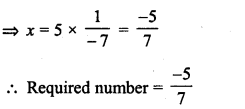Question 7.
By what number should $${ \left( \frac { 1 }{ 2 } \right) }^{ -1 }$$ multiplied so that the product many be equal to $${ \left( \frac { -4 }{ 7 } \right) }^{ -1 }$$
Solution: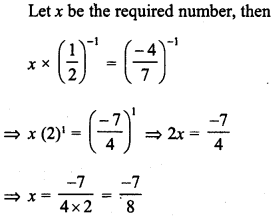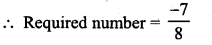Question 8.
By what number should (-15)-1 be divided so that the quotient may be equal to (-5)-1 ?
Solution: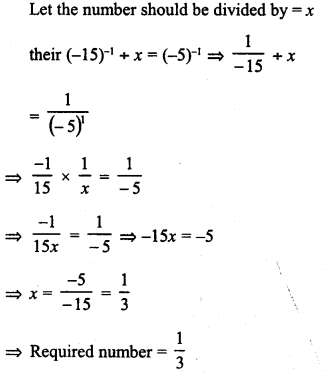Hope given RD Sharma Class 8 Solutions Chapter 2 Powers Ex 2.1 are helpful to complete your math homework.

If you have any doubts, please comment below. Learn Insta try to provide online math tutoring for you.